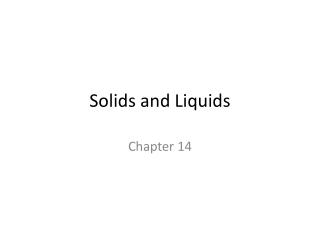DownloadDownload PresentationSolids and Liquids

# Solids and Liquids

Download Presentation## Solids and Liquids

- - - - - - - - - - - - - - - - - - - - - - - - - - - E N D - - - - - - - - - - - - - - - - - - - - - - - - - - -
##### Presentation Transcript

1. Solids and Liquids Chapter 14

2. Intermolecular forces Intramolecular forces The forces that occur among molecules that cause them to aggregate to form a solid or a liquid • Bonding forces that hold atoms together within a molecule

3. Intermolecular forces • Dipole-dipole attraction- molecules with dipole moments can attract each other by lining up so that the positive and the negative ends are close to each other.

4. Hydrogen bonding- hydrogen is bound to two highly electronegative atoms, such as nitrogen, oxygen or fluorine.

5. London dispersion forces- the forces that exist among noble gas atoms and nonpolar molecules

6. Solutions Chapter 15

7. Soluble vs. Insoluble Solubility (or Soluble) Insolubility (or insoluble) Incapable of being dissolved within a given solution • Something that is capable of being dissolved in a solvent

8. Solubility Rules

9. Examples • State which of the following compounds are soluble and which are not • KBr = • PbCO3 = • BSO3 = • zinc hydroxide =

10. KBr = Soluble • PbCO3 = Insoluble • BSO3=Insoluble • zinc hydroxide = Insoluble

11. Types of solutions • Solution-a homogeneous mixture • Solvent-the largest substance that is present within the solution • Solutes-the smallest substance within the solution • Aqueous solution-solutions with water as a solvent • Saturated-a solution that contains as much solute as will dissolve at the temperature • Unsaturated- a solution that has not reached the limit of solute that will dissolve • Concentrated-a large amount of solute dissolved • Dilute-a relatively small amount of solute is dissolved • Supersaturated- a solution that contains more dissolved material than could be dissolved by the solvent under normal circumstances

12. Molarity vs Molality Molarity Molality The amount of substance of solute, divided by the mass of solvent. The number of moles of solute divided by the number of kilograms of solvent Moles of solute = mol Kg of Solution kg • (M) • Describes the amount of solute in moles and the volume of the solution in liters. • The number of moles of solute per volume of solution in Liters • Moles of Solute = mol Liters of Solution L

13. Example of calculating Molarity • Calculate the molarity of a solution prepared by dissolving 11.5 g of solid NaOH in enough water to make 1.50L of solution. • Step 1: calculate the number of moles in NaOH • 11.5g x 1mol = 0.288moles 40 g • Step 2: divide number of moles by the number of Liters • 0.288moles = 0.192M 1.50 L

14. Example of calculating Molality • What is the molality of a solution made from 2.4 moles of NaCl and 0.80 kg of water? • Divide number of moles by the number of kilograms: • 2.4 moles = 3.0m 0.80 kg

15. Net Ionic Equation • Ionic equations are used to describe the chemical reaction while also clearly indicating which of the reactants and products exist primariy as ions in aqueous solutions.

16. Rules for writing net ionic equations • Start with a balanced molecular equation (meaning: an equation that shows the chemical formulas of all reactants and products, but not their physical states) • Break all soluble strong electrolytes (compounds with (aq) beside them into their ions * indicate the correct formula and charge of each ion * indicate the correct number of each ion * write (aq) after each ion • Bring down all compounds with (s), (l), or (g) uncharged

17. For example Use the following equations to answer the question: 2Na3PO4(aq) + 3CaCl2(aq) 6NaCl(aq) + Ca3(PO4)2(s) Net ionic equation: 6Na+ (aq) + 2PO4-3(aq) + 3Ca+2(aq) + 6Cl-(aq) 6Na+(aq) +6Cl-(aq) + Ca3(PO4)2(s)